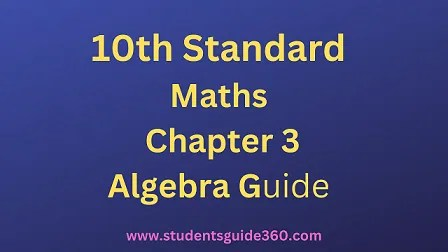# 10th Maths Chapter 3 Algebra Exercise 3.11 Guide

10th Standard Maths Chapter 3 Algebra Samacheer Kalvi Guide Exercise 3.11 Book Back Answers Solutions. TN 10th SSLC Samacheer Kalvi Guide. 10th All Subject Guide – Click Here. Class 1 to 12 All Subject Book Back Answers – Click Here## 10th Maths Chapter 3 Algebra Exercise 3.11 Guide

### 1. Solve the following quadratic equations by completing the square method

• (i) 9×2 – 12x + 4 = 0
• (ii) 5x+7/x−1 = 3x + 2

Solution:### 2. Solve the following quadratic equations by formula method

• (i) 2×2 – 5x + 2 = 0
• (ii) √2f^2 – 6f + 3√2=0
• (iii) 3y2 – 20y – 23 = 0
• (iv) 36y2 – 12ay + (a2 – b2) = 0

Solution:
(i) 2×2 – 5x + 2 = 0
The formula for finding roots of a quadratic equation ax2 + bx + c = 0 is### 3. A ball rolls down a slope and travels a distance d = t2 – 0.75t feet in t seconds. Find the time when the distance travelled by the ball is 11.25 feet.

Solution:
Distance d = t2 – 0.75 t,
Given that d = 11.25 = t2 – 0.75 t.
t2 – 0.75t – 11.25 = 0